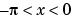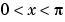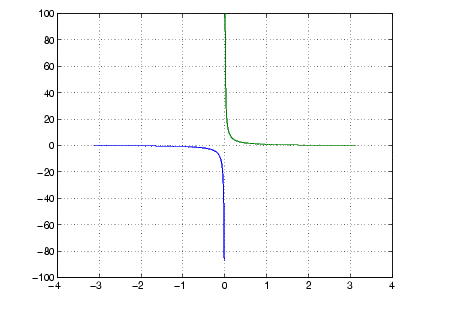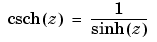MATLAB Function Referencecsch

Hyperbolic cosecant

Syntax

• ```Y = csch(x)
```

Description

The `csch` function operates element-wise on arrays. The function's domains and ranges include complex values. All angles are in radians.

```Y = csch(x) ``` returns the hyperbolic cosecant for each element of `x`.

Examples

Graph the hyperbolic cosecant over the domainsand.

• ```x1 = -pi+0.01:0.01:-0.01;
x2 = 0.01:0.01:pi-0.01;
plot(x1,csch(x1),x2,csch(x2)), grid on```

Definition

The hyperbolic cosecant can be defined as

•Algorithm

`csch` uses FDLIBM, which was developed at SunSoft, a Sun Microsystems, Inc. business, by Kwok C. Ng, and others. For information about FDLIBM, see http://www.netlib.org.

See Also

`acsc`, `acsch`, `csc`

© 1994-2005 The MathWorks, Inc.